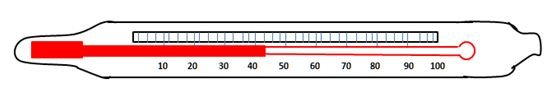# Definition Of Thermometer

## Definition Of Thermometer

Thermometer is a device used for measuring the temperature.

• Different types of thermometers are used for different purposes.
1. Example: Clinical thermometer is used to measure the human body temperature Six�s maximum and minimum thermometer is used to measure the temperature of atmosphere.
• Different scales are used in different thermometers for measuring the temperature.
1. Example: Centigrade Scale (100 divisions will be there on this scale. Lowest is 00 and highest is 1000)
• Fahrenheit scale (180 divisions will be there on this scale. Lowest is 320 and highest is 2120).
• Generally liquid metal mercury is used in thermometers. It expands (mercury thread) as the temperature rises and contracts (come close) when the temperature is decreased.
• It consists of a glass tube on which the required scale is designed.
• Every thermometer depends on some property of material that changes with the temperature.
• All the thermometers agree at the fixed point (Freezing point and boiling point).

#### Example

What temperature is shown by the following thermometer reading?A. 500C
B. 800C
C. 420C
D. 300C
Some thermometer use materials whose electrical properties vary with the temperature.

1. Example: Thermistor thermometer, Thermo couple thermometers.

Thermistor is a device which becomes a much better electrical conductor when its temperature rises.

#### Example

Which property is used in mercury � in � glass thermometer?.
A. Electrical conduction.
B. Electrical insulation.
C. Expansion Mercury.

Step 1: Electrical conduction property is used in Thermistor type thermometer, so the choice "A" is wrong.
Step 2: Electrical insulation property will not be used in any thermometer.
Step 3: Expansion of Mercury on heating is the property which is used in mercury-in-glass thermometer.

Related terms: Temperature | Heat.